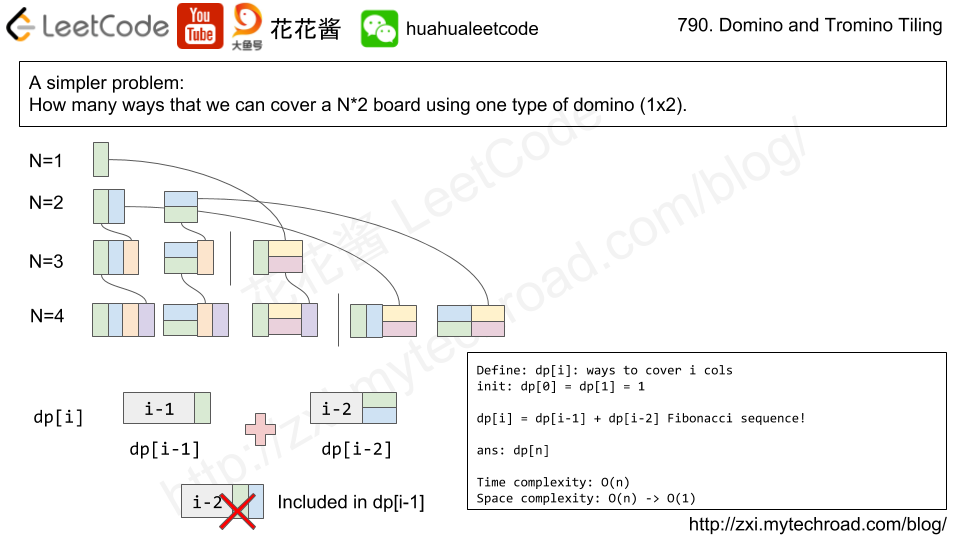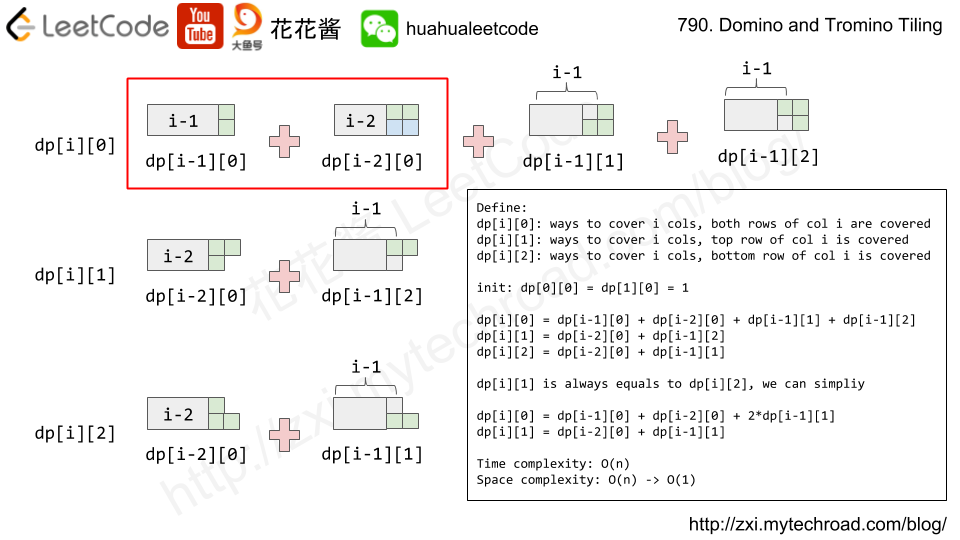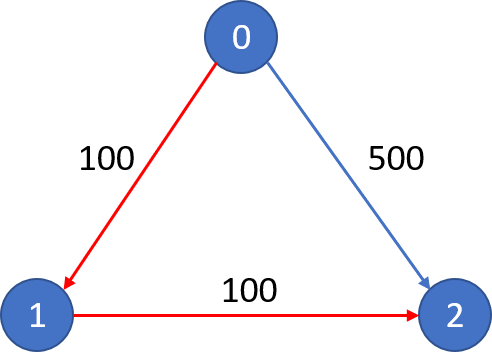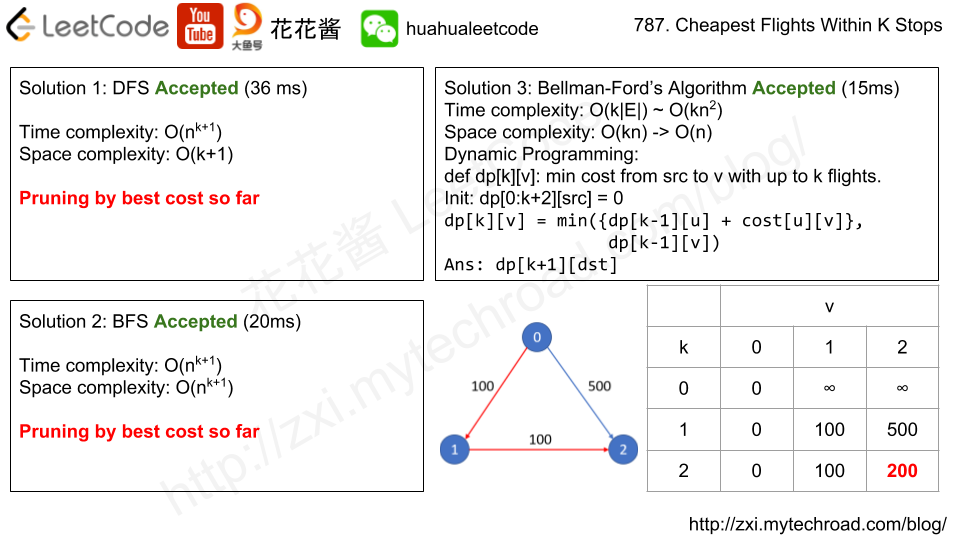Press "Enter" to skip to content

# Posts published in February 2018

We have two types of tiles: a 2×1 domino shape, and an “L” tromino shape. These shapes may be rotated.

Given N, how many ways are there to tile a 2 x N board? Return your answer modulo 10^9 + 7.

(In a tiling, every square must be covered by a tile. Two tilings are different if and only if there are two 4-directionally adjacent cells on the board such that exactly one of the tilings has both squares occupied by a tile.)# Idea: DP

dp[i]: ways to cover i cols, both rows of i-th col are covered
dp[i]:  ways to cover i cols, only top row of i-th col is covered
dp[i]:  ways to cover i cols, only bottom row of i-th col is covered

# Solution 1: DP

Time complexity: O(N)

Space complexity: O(N)

# Solution 2: DP

Another way to think about this problem

define: dp[i] ways to completely covert the i*2 board.

## C++

S and T are strings composed of lowercase letters. In S, no letter occurs more than once.

S was sorted in some custom order previously. We want to permute the characters of T so that they match the order that S was sorted. More specifically, if x occurs before y in S, then x should occur before y in the returned string.

Return any permutation of T (as a string) that satisfies this property.

Note:

• S has length at most 26, and no character is repeated in S.
• T has length at most 200.
• S and T consist of lowercase letters only.

Solution 1: HashTable + Sorting

1. Store the order of char in a hashtable
2. Sort the string based on the order

Time complexity: O(nlogn)

Space complexity: O(128)

You are playing a simplified Pacman game. You start at the point (0, 0), and your destination is (target, target). There are several ghosts on the map, the i-th ghost starts at (ghosts[i], ghosts[i]).

Each turn, you and all ghosts simultaneously *may* move in one of 4 cardinal directions: north, east, west, or south, going from the previous point to a new point 1 unit of distance away.

You escape if and only if you can reach the target before any ghost reaches you (for any given moves the ghosts may take.)  If you reach any square (including the target) at the same time as a ghost, it doesn’t count as an escape.

Return True if and only if it is possible to escape.

Note:

• All points have coordinates with absolute value <= 10000.
• The number of ghosts will not exceed 100.

Solution: Greedy / Math

You can escape if and only if no ghosts can reach target before you. Just need to compare the Manhattan distance.

C++

Time complexity: O(|ghost|)

Java

Python3

1. 出现数字3,4,7一定不合法
2. 新字符串 == 原来字符串不合法

X is a good number if after rotating each digit individually by 180 degrees, we get a valid number that is different from X. A number is valid if each digit remains a digit after rotation. 0, 1, and 8 rotate to themselves; 2 and 5 rotate to each other; 6 and 9 rotate to each other, and the rest of the numbers do not rotate to any other number.

Now given a positive number N, how many numbers X from 1 to N are good?

Note:

• N  will be in range [1, 10000].

Solution 1: Brute Force

Time complexity: O(nlogn)

C++

Bit  Operation

There are n cities connected by m flights. Each fight starts from city u and arrives at v with a price w.

Now given all the cities and fights, together with starting city src and the destination dst, your task is to find the cheapest price from src to dst with up to k stops. If there is no such route, output -1.Note:

• The number of nodes n will be in range [1, 100], with nodes labeled from 0 to n - 1.
• The size of flights will be in range [0, n * (n - 1) / 2].
• The format of each flight will be (src, dst, price).
• The price of each flight will be in the range [1, 10000].
• k is in the range of [0, n - 1].
• There will not be any duplicated flights or self cycles.# Solution 1: DFS

w/o prunning TLE

w/ prunning Accepted

# Solution 3: Bellman-Ford algorithm

dp[k][i]: min cost from src to i taken up to k flights (k-1 stops)

init: dp[0:k+2][src] = 0

transition: dp[k][i] = min(dp[k-1][j] + price[j][i])

ans: dp[K+1][dst]

Time complexity: O(k * |flights|) / O(k*n^2)

Space complexity: O(k*n) -> O(n)

w/o space compression O(k*n)

## Python3

Mission News Theme by Compete Themes.